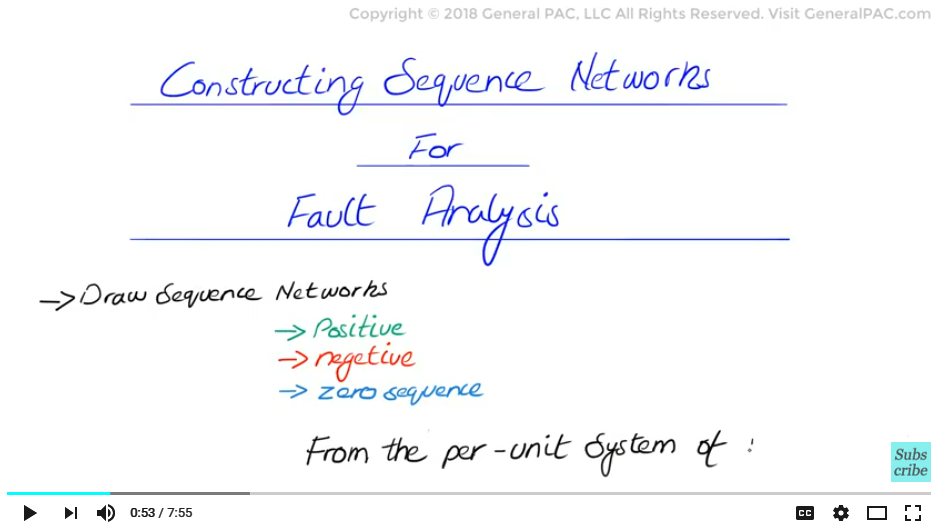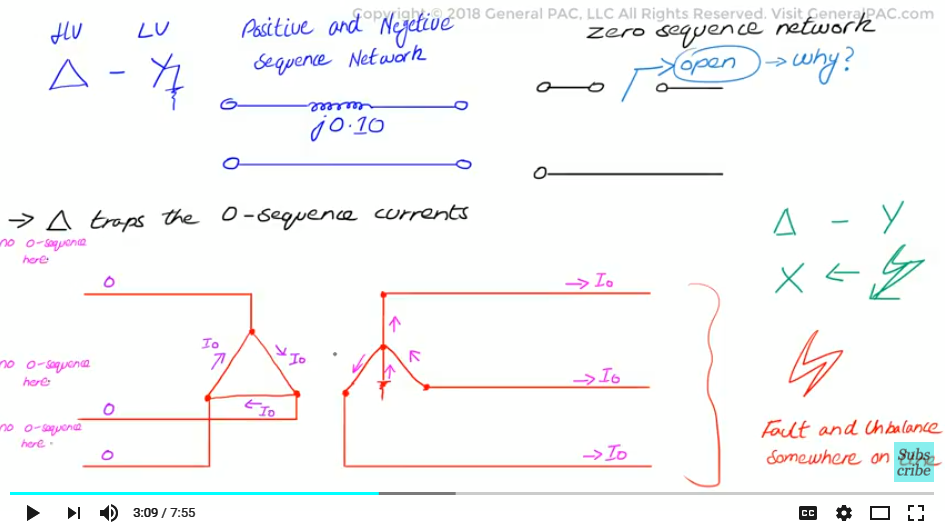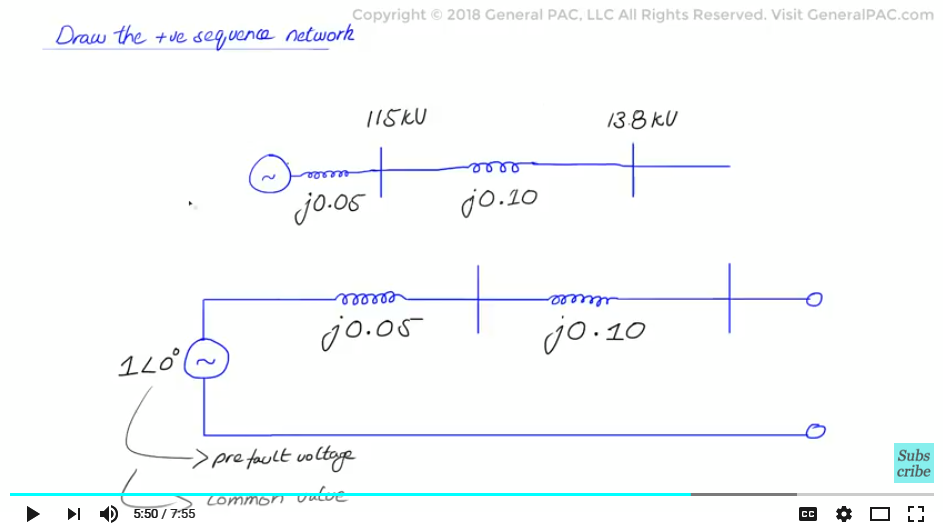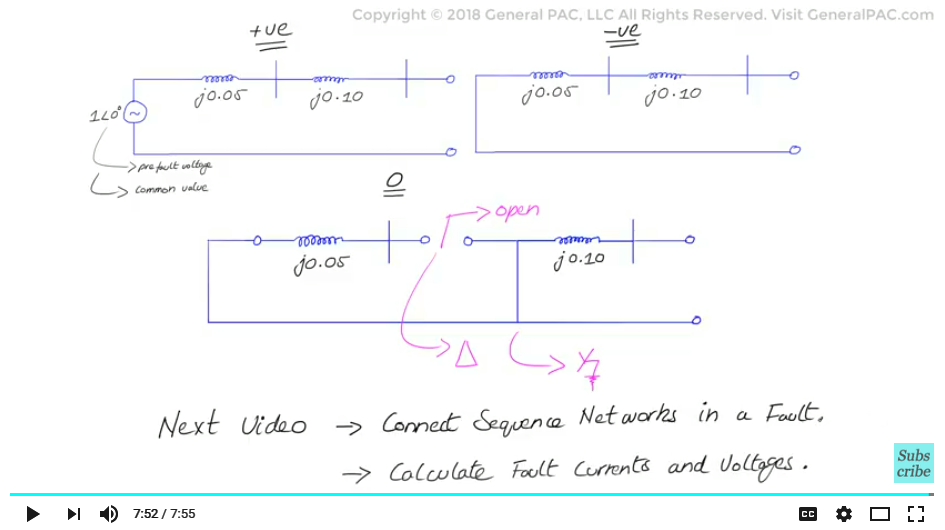If this video was helpful for you, please consider subscribing to GeneralPAC.com and becoming our patreon at patreon.com/generapac. Your donation will allow us to continue making high quality video tutorials free for everyone, everywhere…. Our goal is simple; we make Power Systems intuitive.

Our corporate sponsor for this topic is AllumiaX.com, from Seattle Washington. Contact them for industrial and commercial power system studies like short circuit, coordination, and arc flash studies.

Fault analysis in power systems part 2c, constructing sequence networks for fault analysis, now this is the fourth video in the series of fault analysis in power systems and in this video we will learn how to draw the sequence networks for each of the positive negative and zero sequence networks from the system that was converted in Part – 2b.So the network diagram that was drawn previously is a loose representation of a positive sequence network which already has the delta wye transformer sequence model that is integrated in it. We haven’t represented the negative sequence or zero sequence network diagrams yet which is going to be the basis of this video. So, because the delta wye transformer connection is the most complex part in the sequence network analysis we are gonna spend most of our time talking about that in this tutorial.

So if the transformer is a delta wye transformer with the neutral of the wye side or the low voltage side being connected solidly grounded then the transformer’s positive and negative sequence networks can just be represented by a single impedance as shown below, however although this is very simple, the zero sequence network will be a little different, the delta side of this zero sequence network is treated as an open circuit. Now why is the delta side or the high voltage side treated as an open circuit? The intuitive explanation will require another topic altogether but the basic example is that the delta winding traps the zero sequence current and does not allow it to flow. Meaning the zero sequence current on either direction of the transformer, meaning if there is a high voltage fault the zero sequence current cannot be reflected on the low voltage side or if there is a low voltage fault then the zero sequence current cannot be reflected on the high voltage side.

Now refer to the figure below which shows a low voltage fault being reflected on the high voltage side now these are only zero sequence currents as you can see there is a fault somewhere out on the line and there are zero sequence currents that are flowing through that fault but we don’t see any zero sequence current on the high voltage side which is the delta side of the transformer.Now the second point here is that the wye side of the transformer can be represented by the series impedance Zt connected to ground at one end only because the neutral terminal of the low voltage side of the transformer this is the wye side is connected to ground. So the ground connection will cause the zero sequence current to flow into ground and allow the fault to circulate but remain on the low voltage side only because the zero sequence currents will not be reflected on the high voltage side as we discussed earlier because of the delta connection. Which is modeled as an open circuit for the zero sequence network. This is only true for the zero sequence current. Right because the transformer will have a negative sequence current reflected on the high voltage side but it won’t have a zero sequence current, now this concept again requires the topic in itself so we will keep this brief and continue with our example.

Now another thing to keep in mind that different transformer connections will require different zero sequence network diagrams although the positive and negative sequence networks will remain the same zero sequence network is going to be different base of the transformer connection. Now this is true for both two winding transformers as well as three winding transformers.

So now lets draw the positive sequence network right, in the positive sequence network which is drawn we haven’t covered this in part 2b but the generator’s voltage source per unit values will be one per unit at the angle of zero degrees now this is fairly simple because we conveniently selected 115 kV as the base voltage which was same voltage as our generator source. The voltage source is also sometimes called pre-fault voltage selecting 1 per unit at 0 degrees is a very common value for fault analysis. Now this short circuit impedance of the generator j.05 per unit is added in series next. A line is drawn to mark the 115 kV bus and then the positive sequence representation of the delta wye transformer is drawn with a series impedance of j.10 per unit, a line then is also drawn for the 13.8kV bus. Now remember the voltage this line is only for the voltage references and is meant to be a guide. It is not meant to reflect actual voltage son the circuit, so that was the positive sequence network.Now let’s draw the negative sequence network. For the negative sequence network it is essentially the same as the positive sequence network with the exception that there is no voltage source. This is because the generator does not produce negative sequence currents and therefore does not injects negative sequence currents into the system during a faulted condition, but negative sequence current will still flow through the winding for a balanced and unbalanced fault. So we still represent j.05 per unit but we don’t represent the actual voltage source of 1 per unit at 0 degrees. So it is very similar to the positive sequence network diagram.

Now we move towards the zero sequence network diagram, now the zero sequence network diagram just like the negative sequence network diagram, there is no voltage source, there is the same generator impedance of j.05 per unit, then there is a line to indicate 115 kV bus and then we reach the transformer, pick up the zero sequence model for the delta wye transformer that was discussed in the video earlier and insert it into the network with the impedance of .10 per unit, and then there is a line to indicate the 13.8 KV bus and the network is then complete, now notice that for the network diagram of the transformer there is an open on the high voltage side of the transformer which represents the delta and on the low voltage side there is a short immediately before the .05 per unit impedance, that short represents the low voltage wye side of the transformer connected to ground.

Now in the next video we will show how we can connect these three individual sequence networks in the event of a fault and use them to calculate the fault currents and voltages quite easily. So please join us for part 3 in the next video. Thank You.#### Greetings from the GeneralPAC Team!

We make high-quality Power Systems Video Tutorials on complex topics that are free and open to everyone!  Thank you so much for supporting us through Patreon so can continue doing good and valuable work.

What is Patreon and why do we use it?

Patreon is a fantastic portal that allows our fans and community to make monthly contribution (like Netflix subscription) so we can continue creating high-quality power systems video tutorials. In return, you get access to incredible perks like voting on future topics, getting your questions answered, access to VIP Q/A webinars with the creators of GeneralPAC, and much more! We THANK YOU for supporting us

Why do we need your support?

An incredible amount of time and effort is needed to develop high-quality video tutorials. Each video (Part 1 for example) takes approximately 10 hours to complete which includes learning the concept ourselves, brainstorming creative ways to teach and explain the concepts, writing the script, audio recording, video recording, and editing. It's no wonder why Hundreds-of-Thousands of people have watched, liked, subscribed, and left positive comments on Youtube channel. Your support truly makes all the difference.